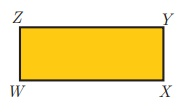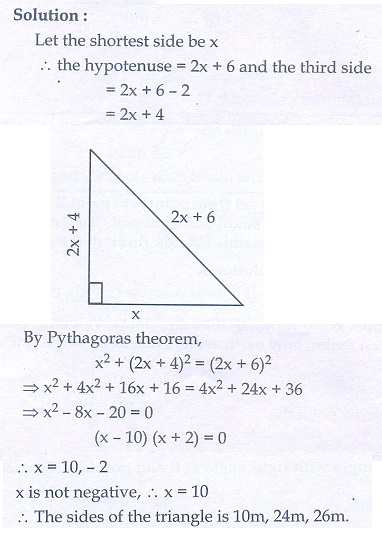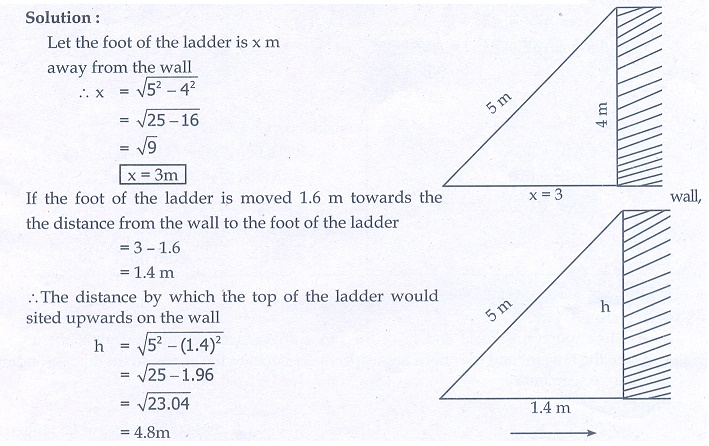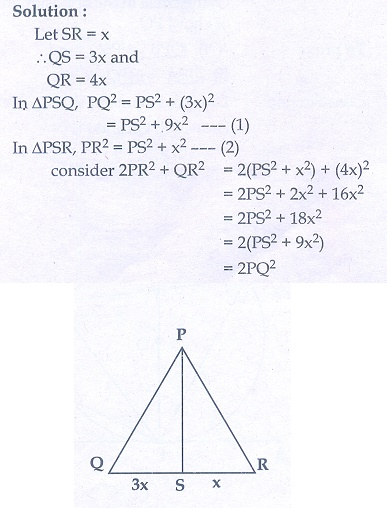Home | | Maths 10th Std | Exercise 4.3: Pythagoras Theorem

# Exercise 4.3: Pythagoras Theorem

Maths Book back answers and solution for Exercise questions - Mathematics : Geometry: Pythagoras Theorem: Exercise Problem Questions with Answer

Exercise 4.3

1. A man goes 18 m due east and then 24 m due north. Find the distance of his current position from the starting point?2. There are two paths that one can choose to go from Sarah’s house to James house. One way is to take C street, and the other way requires to takeA street and then B street. How much shorter is the direct path along C street? (Using figure).3. To get from point A to point B you must avoid walking through a pond. You must walk 34 m south and 41 m east. To the nearest meter, how many meters would be saved if it were possible to make a way through the pond?4. In the rectangle WXYZ, XY+YZ=17 cm, and XZ+YW=26 cm. Calculate the length and breadth of the rectangle?5. The hypotenuse of a right triangle is 6 m more than twice of the shortest side. If the third side is 2 m less than the hypotenuse, find the sides of the triangle.6. 5 m long ladder is placed leaning towards a vertical wall such that it reaches the wall at a point 4m high. If the foot of the ladder is moved 1.6 m towards the wall, then find the distance by which the top of the ladder would slide upwards on the wall.7. The perpendicular PS on the base QR of a ΔPQR intersects QR at S, such that QS = 3 SR. Prove that 2PQ2  = 2PR2 +QR8. In the adjacent figure, ABC is a right angled triangle with right angle at B and points DE trisect BC. Prove that 8AE 2 = 3AC 2 + 5AD21. 30 m

2. 1 mile

3. 21.74 m

4. 12 cm, 5 cm

5. 10 m, 24 m, 26 m

6. 0.8 m

Tags : Problem Questions with Answer, Solution | Mathematics , 10th Mathematics : UNIT 4 : Geometry
Study Material, Lecturing Notes, Assignment, Reference, Wiki description explanation, brief detail
10th Mathematics : UNIT 4 : Geometry : Exercise 4.3: Pythagoras Theorem | Problem Questions with Answer, Solution | Mathematics